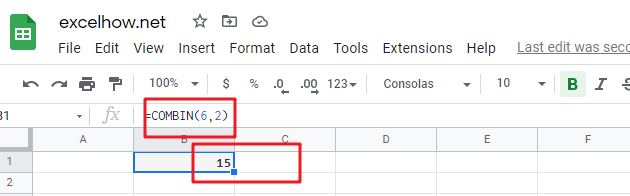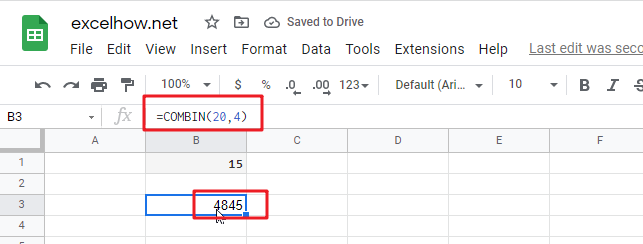# ExcelHow

This post will guide you how to use Google Sheets COMBIN function with syntax and examples.

## Description

The Google Sheets COMBIN function returns the number of combinations for a given number of items. And you can use the COMBIN function to determine the total possible number of groups for a given number of items.

For example, assuming that you have 6 objects(a,b,c,d,e,f) and you can get 15 different combinations of 2 objects , the combinations are as follows:

`ab ac ad ae af bc bd be bf cd ce cf de df ef`

The purpose of this function is used to get number of combinations without repetitions in google sheet and it’s returned value is a number of combinations as an integer.

The COMBIN function is a build-in function in Google Sheets and it is categorized as a MATH function.

## Syntax

The syntax of the COMBIN function is as below:

=COMBIN (number, number_chosen)

Where the COMBIN function arguments are:

• number – This is a required argument. . The number of items. It must be greater than 0, and it also must be greater than number_chosen argument.
• number_chosen – This is a required argument. The number of combinations that you want to.

Note:

• If either the number argument or the number_chosen argument is less than 0, then the COMBINA function will return the #NUM! Error.
• If any arguments are non-numeric, this function will return #VALUE! Error.
• If any arguments are integer values and will be truncated.
• if you want to calculate the number of combinations for a given number of items (with repetitions), you can use the COMBINA function.

## Google Sheets COMBIN Function Examples

The below examples will show you how to use google sheets COMBIN Function to get the number of combinations for a given number objects from a set (in any order).

1# to get the number of combinations for 6 and 2(in any order), enter the following formula in Cell B1.

`=COMBIN(6,2)`2# to get the number of combinations for 20 and 4(in any order), enter the following formula in Cell B2.

`=COMBIN(20,4)`